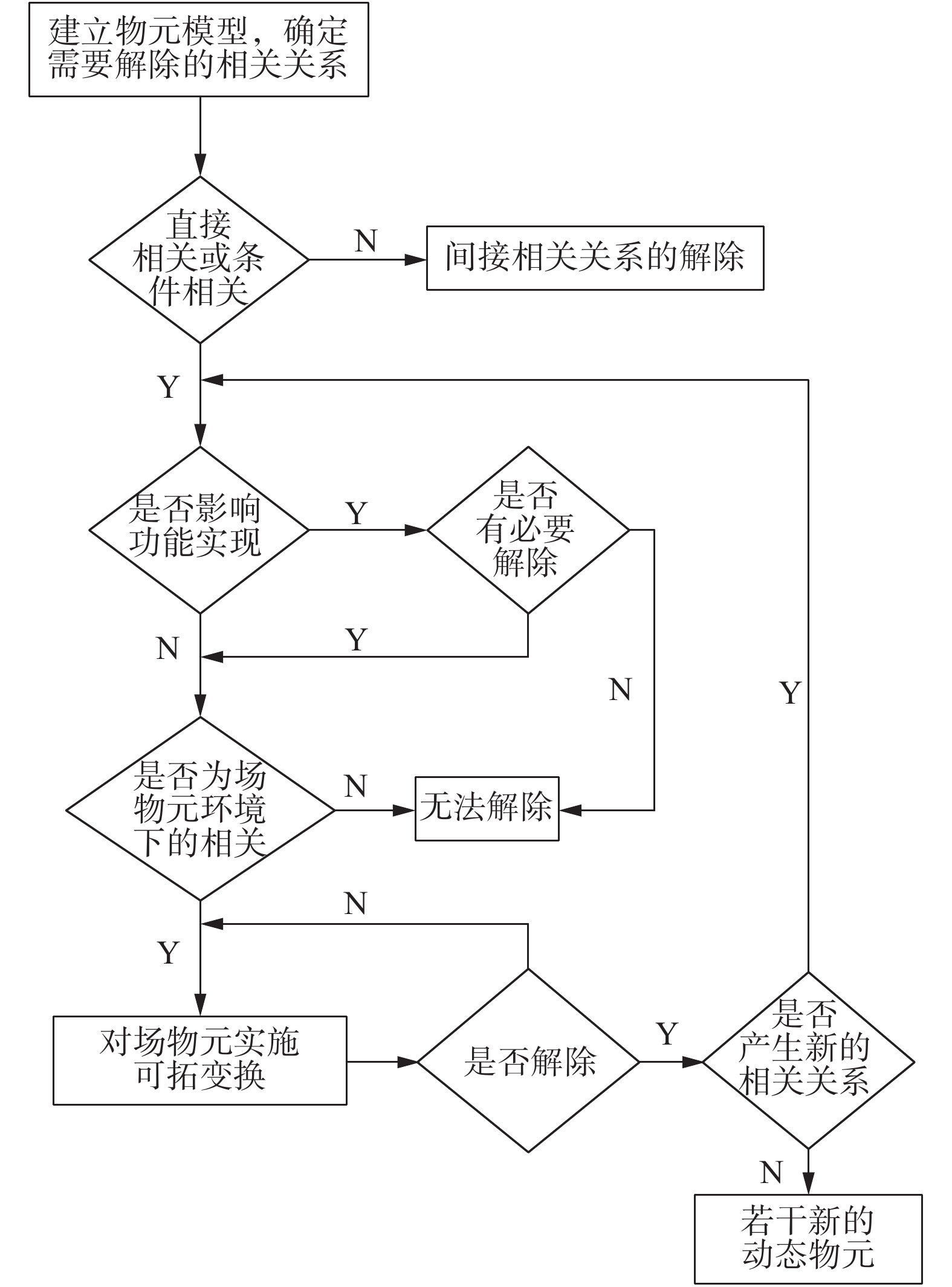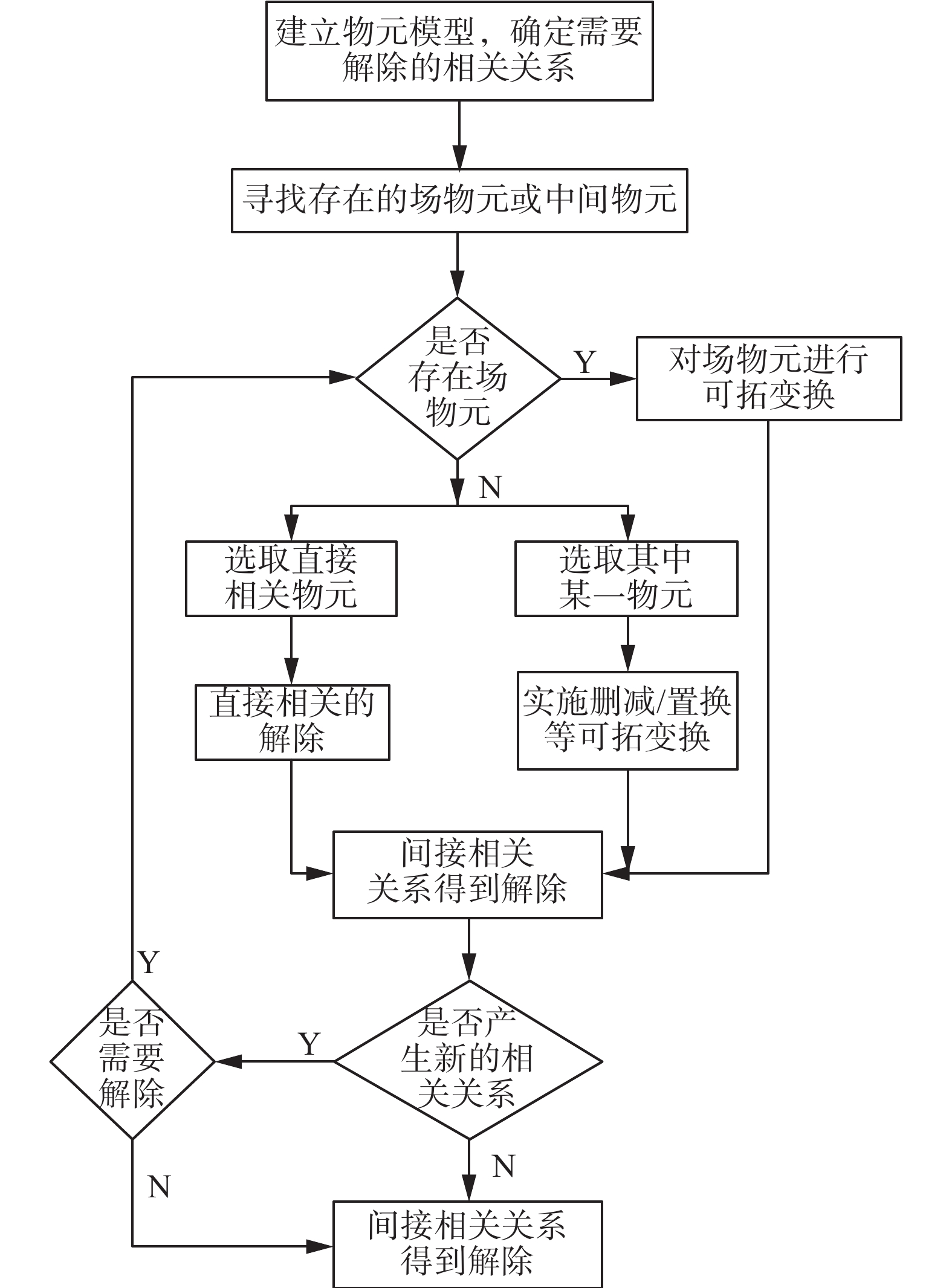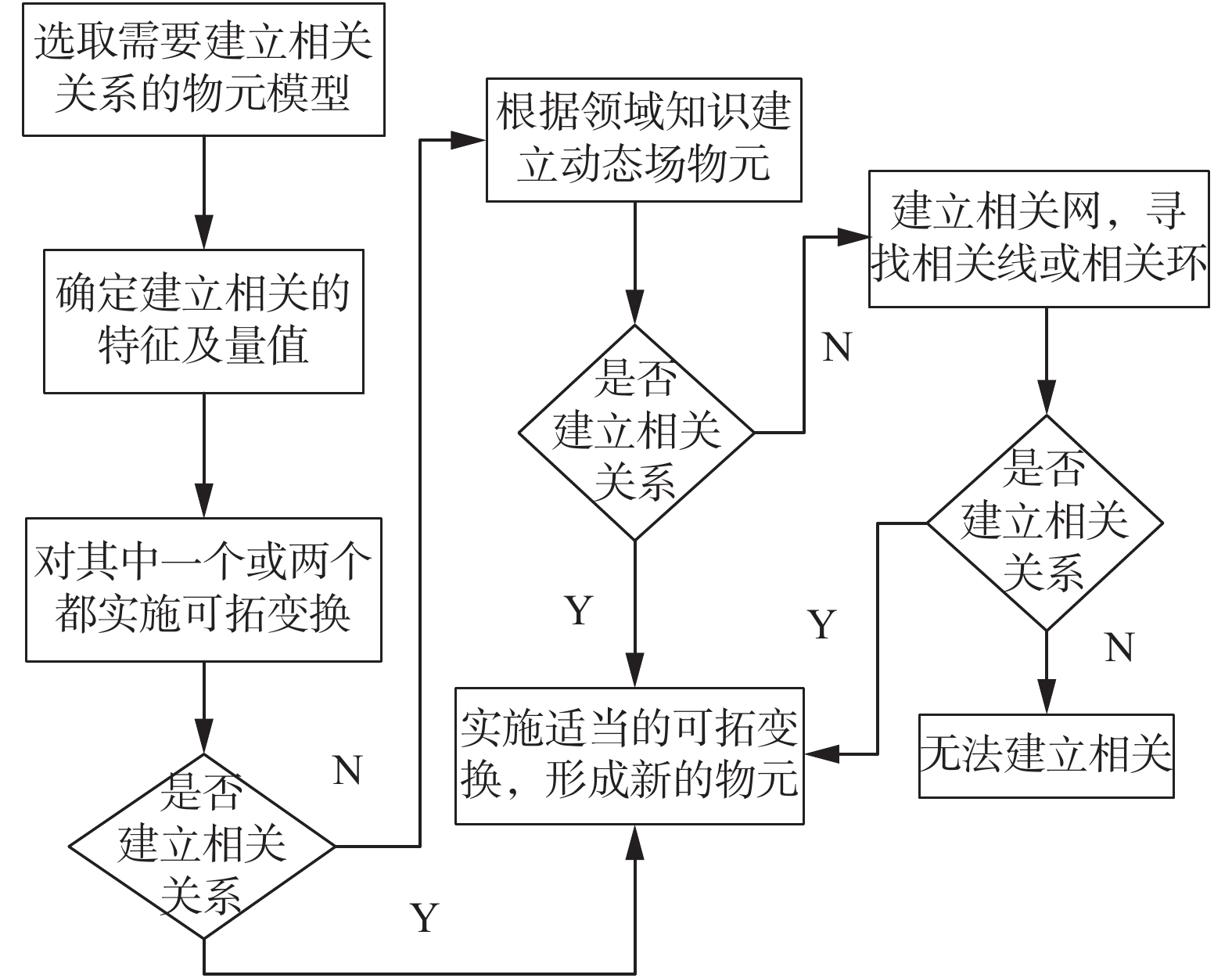﻿ 可拓学中相关关系的变换方法研究
«上一篇文章快速检索 高级检索

 智能系统学报2019, Vol. 14Issue (4): 619-626  DOI: 10.11992/tis.2018110270

### 引用本文LI Wenjun, YANG Chunyan, TANG Long, et al. Research on the transformation method for the correlation relation in extenics[J]. CAAI Transactions on Intelligent Systems, 2019, 14(4): 619-626. DOI: 10.11992/tis.201811027.### 文章历史

1. 广东工业大学 可拓学与创新方法研究所，广东 广州 510006;
2. 广东工业大学 机电工程学院，广东 广州 510006;
3. 广州市德百顺电气科技有限公司，广东 广州 510700

Research on the transformation method for the correlation relation in extenics
LI Wenjun 1,2,3, YANG Chunyan 1,2, TANG Long 1,2, ZHANG Wenying 3, ZHONG Jianhua 3
1. Research Institute of Extenics and Innovation Methods, Guangdong University of Technology, Guangzhou 510006, China;
2. School of Electromechanical Engineering, Guangdong University of Technology, Guangzhou 510006, China;
3. Guangzhou Deposon Electric Technology Co., Ltd., Guangzhou 510700, China
Abstract: In this study, a method for changing the correlative relations among matters and affairs was established to explore the theory and method for solving contradiction problems by studying the correlation relations among matters and affairs based on the theories of correlation analysis method, extension transformation, TRIZ, and other theories in extenics in combination with the practical engineering experience. Using "lamp" as an example, the proposed method was applied to the innovation of the lamp products and the analysis and solution of the filament-related technical problems. Using the established methods, an effective new product creativity was established, i.e., a lamp that can change color based on the mood of user, and an approach was developed to enhance the properties of the manufactured product, verifying the feasibility and effectiveness of the proposed method in practical applications.
Key words: extenics    dynamic    correlation relations    correlation analysis    transform    force to establish    force to dissolve    weaken    matter-element field

TRIZ理论作为一种创新理论，在工程运用以及专利分析等领域起到重要的作用[9-12]。虽然没有研究事物之间的相关性，但是其中某些理论和方法仍然可以用来作为研究相关关系的理论依据。文献[13-18]中对物−场模型的研究是用于解决实际应用中的物理矛盾问题，文献将可拓学与TRIZ理论进行了有效的结合，利用可拓学的思维逻辑对TRIZ理论中的40条原理进行了阐述，为可拓学与TRIZ理论在某些方面的结合研究提供了一定的基础。本文将两者结合，通过对两者各自的某些优点进行融合和创新，将更有利于对相关关系的变换方法进行研究。

1 相关分析原理与可拓变换方法

1.1 相关分析原理

1.1.1 物元相关的定义

1.1.2 物元相关规则

1.2 动态物元之间的可拓变换和传导变换

2 动态物元间相关关系的变换方法

2.1 强化和弱化动态物元之间相关关系的方法

 $\frac{{{v_2}({t_2}) - {v_2}({t_1})}}{{{v_1}({t_2}) - {v_1}({t_1})}} = \frac{{{f_1}({v_1}({t_2})) - {f_1}({v_1}({t_1}))}}{{{v_1}({t_2}) - {v_1}({t_1})}} > 0$ (1)

 $\frac{{{v_2}({t_2}) - {v_2}({t_1})}}{{{v_1}({t_2}) - {v_1}({t_1})}} = \frac{{{f_1}({v_1}({t_2})) - {f_1}({v_1}({t_1}))}}{{{v_1}({t_2}) - {v_1}({t_1})}} < 0$ (2)

1) 根据实际问题建立动态物元模型，一般至少是3个动态物元之间的相关；

2) 根据公式计算相关关系的相关度，并确定其正负性；

3) 确定这些相关关系的相对强弱性：

①若定义3中的两个动态物元M1(t)和M2(t)分别与动态物元M(t)=(O(t)，cv(t))相关，即存在v(t)=f(v1(t)，v2(t))且可导，则当 $\left| {\dfrac{{\partial f}}{{\partial \left( {{v_1}\left( t \right)} \right)}} \cdot \dfrac{{\partial \left( {{v_1}\left( t \right)} \right)}}{{\partial \left( t \right)}}} \right| > \left| {\dfrac{{\partial f}}{{\partial \left( {{v_2}\left( t \right)} \right)}} \cdot \dfrac{{\partial \left( {{v_2}\left( t \right)} \right)}}{{\partial \left( t \right)}}} \right|$ 时认为动态物元M1(t)与动态物元M(t)为相对强相关，同时，认为动态物元M2(t)与动态物元M(t)为相对弱相关；当 $\left| {\dfrac{{\partial f}}{{\partial \left( {{v_1}\left( t \right)} \right)}} \cdot \dfrac{{\partial \left( {{v_1}\left( t \right)} \right)}}{{\partial \left( t \right)}}} \right| < \left| {\dfrac{{\partial f}}{{\partial \left( {{v_2}\left( t \right)} \right)}} \cdot \dfrac{{\partial \left( {{v_2}\left( t \right)} \right)}}{{\partial \left( t \right)}}} \right|$ 时认为动态物元M1(t)与动态物元M(t)为相对弱相关，同时，认为动态物元M2(t)与动态物元M(t)为相对强相关。

②若①中的相关函数v(t)=f(v1(t)，v2(t))为不可导、离散函数和间断函数中的一种时，则根据实际情况结合领域专业知识对动态物元M1(t)和M2(t)分别与动态物元M(t)相关的相对强弱性进行判断。

4) 理论结合实际，确定动态物元之间需要强化和弱化的相关关系；

5) 依据4)中确定的变换思路，对动态物元进行可拓变换，实现相关关系的强化与弱化，并通过增强理想的相关影响，减弱非理想的相关影响，来实现消除非理想相关产生负面影响，实现问题解决的目的。

2.2 动态物元之间相关关系解除的方法

 ${{{M}}_{{f}}} = \left[ {\begin{array}{*{20}{c}} {{{{O}}_{{f}}},}&{{{{c}}_{{{f}}1}},}&{{{{v}}_{{{f}}1}}}\\ {}&{{{{c}}_{{{f}}2}},}&{{{{v}}_{{{f}}2}}}\\ {}& \vdots & \vdots \end{array}} \right] = \left( {\begin{array}{*{20}{c}} {{{{O}}_{{f}}},}&{{{{C}}_{{f}}},}&{{{{V}}_{{f}}}} \end{array}} \right)$

 $\begin{split} {{{M}}_{{f}}}\left( {{t}} \right) = & \left[ {\begin{array}{*{20}{c}} {{{{O}}_{{f}}}\left( {{t}} \right),}&{{{{c}}_{{{f}}1}}\left( {{t}} \right),}&{{{{v}}_{{{f}}1}}\left( {{t}} \right)}\\ {}&{{{{c}}_{{{f}}2}}\left( {{t}} \right),}&{{{{v}}_{{{f}}2}}\left( {{t}} \right)}\\ {}& \vdots & \vdots \end{array}} \right] = \\ & \left( {\begin{array}{*{20}{c}} {{{{O}}_{{f}}}\left( {{t}} \right),}&{{{{C}}_{{f}}}\left( {{t}} \right),}&{{{{V}}_{{f}}}\left( {{t}} \right)} \end{array}} \right) \end{split}$

2.2.1 直接相关与条件相关关系解除的一般步骤

1)从实际出发，建立动态物元模型并确定动态物元之间需要解除的相关关系；

2)对1)中确定的动态物元之间的相关关系进行分析，并确定这些相关关系是直接相关还是间接相关；

3)判断这些相关关系是否影响产品功能的实现，如果影响则还需判断该功能是否为必须功能，以及影响的程度。

4)若3)中的两个动态物元之间的相关关系是某个动态场物元的作用下形成，则对该动态场物元实施可拓变换，使两个动态物元发生传导变换，进而使得两个动态物元之间的相关关系发生变化。

5)一般情况下，4)中对动态场物元实施可拓变换之后，产生的传导变换导致两个动态物元之间的相关关系发生变化时，需要重新对两个动态物元本身以及两者之间的相关关系进行分析。Download: 图 1 直接相关关系解除流程图 Fig. 1 Direct correlation dissolves flow chart
2.2.2 间接相关关系解除的一般步骤

1)从实际出发，建立动态物元模型并确定动态物元之间需要解除的相关关系；

2)对1)中确定的相关关系进行分析，并从中找出需要解除的间接相关关系，找到两者之间存在的相关的中间动态物元或动态场物元；

3)若两个动态物元通过动态场物元间接相关，则找到使得两动态物元相关的动态场物元的特征(即某种作用力或作用效果)，对该特征对应的分物元进行删减变换或扩缩变换，使得该动态场物元对应的特征无法产生作用，或者该作用发生变化，导致两动态物元不再相关；

①对两个动态物元之间的其他动态物元实施置换变换或者删减变换等可拓变换，使其中的某些或者某个物元不再相关或者使其相关特征的量值改变，从而使原本相关的某些中间动态物元不再相关，从而打断两个动态物元通过中间动态物元传递相关的媒介，使其不再相关，实现两个动态物元之间相关关系的解除。

②针对两个动态物元之间传递相关关系的直接相关的中间动态物元，运用解除直接相关或者条件相关的方法，对其进行相关关系的解除，从而通过阻止相关关系的传递，实现相关关系的解除。Download: 图 2 间接相关关系解除流程图 Fig. 2 Indirect correlation dissolves flow chart
2.3 动态物元之间相关关系的建立方法

1)从实际出发，建立动态物元模型并确定动态物元之间需要建立的相关关系。

2)确定1)中需要建立的动态物元之间的相关关系的相关特征及其量值。

3)对其中至少一个需要建立相关关系的特征实施可拓变换，使特征的量值之间产生某种函数关系，此时便在实施可拓变换的条件下实现两个动态物元之间相关关系的建立。

4)最后根据建立的相关关系，进行适当的可拓变换，形成若干新的动态物元，便可以通过强制建立相关的方式实现具体问题的解决。Download: 图 3 动态物元间相关关系建立流程图 Fig. 3 Establish flow chart for dynamic matter-element correlation
3 实例分析

 $\begin{split} & \qquad \qquad \qquad \qquad \qquad{{M}}({{t}}) = \\ & \left[ \!\!\!\! \!\!{\begin{array}{*{20}{c}} \begin{array}{l} \text{灯泡}{{D}}({{t}}),\\ \\ \\ \\ \\ \\ \\ \end{array}&\begin{array}{l} \text{功率}{{{c}}_1},\\ \text{类型}{{{c}}_2},\\ \text{颜色}{{{c}}_3},\\ \text{灯丝性能}{{{c}}_4},\\ \text{寿命}{{{c}}_5},\\ \text{期望使用时间}{{{c}}_6},\\ \text{亮度}{{{c}}_7},\\ \text{工作电流}{{{c}}_8}, \end{array}&\begin{array}{l} {{{v}}_1}({{t}})\\ {{{v}}_2}\\ {{{v}}_3}({{t}})\\ {{{v}}_4}({{t}})\\ {{{v}}_5}({{t}})\\ {{{v}}_6}({{t}})\\ {{{v}}_7}({{t}})\\ {{{v}}_8}({{t}}) \end{array} \end{array}} \!\!\!\! \!\!\right]\mathop = \limits^\Delta \left[ \!\!\!\! \begin{array}{l} {{{M}}_1}({{t}})\\ {{{M}}_2}({{t}})\\ {{{M}}_3}({{t}})\\ {{{M}}_4}({{t}})\\ {{{M}}_5}({{t}})\\ {{{M}}_6}({{t}})\\ {{{M}}_7}({{t}})\\ {{{M}}_8}({{t}}) \end{array} \!\!\!\!\right] \end{split}$

1)通过强化/弱化相关关系的方法解决灯泡使用时间长导致的灯泡亮度变暗问题。

t2>t1，则有

 $\frac{{{v_{7}}({t_2}) - {v_{7}}({t_1})}}{{{v_{5}}({t_2}) - {v_{5}}({t_1})}} = \frac{{{f_{1}}({v_{5}}({t_2})) - {f_{1}}({v_{5}}({t_1}))}}{{{v_{5}}({t_2}) - {v_{5}}({t_1})}} < {0}$

M5(t)与M7(t)负相关。

t2>t1，则有

 $\frac{{{v_{{01}}}({t_2}) - {v_{{01}}}({t_1})}}{{{v_{7}}({t_2}) - {v_{7}}({t_1})}} = \frac{{{f_{2}}({v_7}({t_2})) - {f_2}({v_7}({t_1}))}}{{{v_7}({t_2}) - {v_7}({t_1})}} > {0}$

M7(t)与M01(t)正相关。

 $\frac{{{f_{2}}\left[ {{f_{1}}({v_{5}}({t_{2}}))} \right] - {f_{2}}\left[ {{f_{1}}({v_{5}}({t_{1}}))} \right]}}{{{f_{1}}({v_{5}}({t_{2}})) - {f_{1}}({v_{5}}({t_{1}}))}} < \frac{{{f_{1}}({v_{5}}({t_{2}})) - {f_{1}}({v_{5}}({t_{1}}))}}{{{v_{5}}({t_{2}}) - {v_{5}}({t_{1}})}}$

t时刻，称M5(t)与M7(t)强相关，M7(t)与b弱相关。

φM7(t)=(灯泡D(t)，亮度c7v7(t))=M7(t)

TφM5(t)=Tφ(灯泡D(t)，寿命c5v5(t))=M5(t)其中，v′5(t)>v5(t);

$T{'}_{\varphi}$ M01(t)=T′φ(房间O01(t)，期望亮度，v′01(t))=M′01(t)其中，v′01(t)<v01(t)。

 ${\varphi _1}{{{M}}_4}\left( {{t}} \right) = \left( {\text{灯泡}{{D}}\left( {{t}} \right),{{{c}}_4},{{{{v'}}}_4}\left( {{t}} \right)} \right) = {{{M'}}_4}\left( {{t}} \right)$

2)通过强制解除相关关系的方法解决灯泡亮度越高使用寿命越短的问题。

 ${\varphi _2}{{{M}}_6}\left( {{t}} \right) = \left( {\text{灯泡}{{D''}}\left( {{t}} \right),{{{c}}_6},{{{{v'}}}_6}\left( {{t}} \right)} \right) = {{{M'}}_6}\left( {{t}} \right)$

3)通过强制建立相关关系的方法进行产品创新。

 ${{{M}}_{{\bf{03}}}}({{t}}) = \left[ \!\!\!\!\!\! \!\!{\begin{array}{*{20}{c}} \begin{array}{l} \text{房间}{{{D}}_{03}}({{t}}),\\ \end{array}&\begin{array}{l} \text{亮度},\\ \text{温度}, \end{array}&\begin{array}{l} {{{v}}_{031}}({{t}})\\ {{v}}{}_{032}({{t}}) \end{array} \end{array}}\!\!\!\!\!\! \!\! \right]\mathop = \limits^\Delta \left[\!\!\!\! \begin{array}{l} {{{M}}_{031}}({{t}})\\ {{{M}}_{032}}({{t}}) \end{array}\!\!\!\! \right]$

 ${{{M}}_{0{\rm{4}}}}({{t}}) = \left[\!\!\!\!\!\!\!\! {\begin{array}{*{20}{c}} \begin{array}{l} {{{D}}_{04}}({{t}}),\\ \\ \\ \\ \\ \end{array}&\begin{array}{l} \text{感应亮度},\\ \text{设定亮度},\\ \text{输出信号}1,\\ \text{感应温度},\\ \text{设定温度},\\ \text{输出信号}2, \end{array}&\begin{array}{l} {{{v}}_{041}}({{t}})\\ {{{v}}_{042}}({{t}})\\ {{{v}}_{043}}({{t}})\\ {{{v}}_{044}}({{t}})\\ {{{v}}_{045}}({{t}})\\ {{{v}}_{046}}({{t}}) \end{array} \end{array}}\!\!\!\!\!\!\!\! \right]\mathop = \limits^\Delta \left[\!\!\!\! \begin{array}{l} {{{M}}_{041}}({{t}})\\ {{{M}}_{042}}({{t}})\\ {{{M}}_{043}}({{t}})\\ {{{M}}_{044}}({{t}})\\ {{{M}}_{045}}({{t}})\\ {{{M}}_{046}}({{t}}) \end{array}\!\!\!\! \right]$

 $此时有：${{{M}}_{031}}({{t}})\mathop {{\widetilde{\to}}}\limits^{{{{M}}_{04}}({{t}})} {{{M}}_{\bf{7}}}({{t}})
 ${{{M}}_{032}}({{t}})\mathop {{\widetilde{\to}}}\limits^{{{{M}}_{04}}({{t}})} {{{M}}_{\bf{1}}}({{t}})$

4 结束语

  杨春燕, 蔡文.可拓学[M]. 北京: 科学出版社, 2014: 43-46, 130-133. (0)  杨春燕.可拓创新方法[M]. 北京: 科学出版社, 2017: 10-103, 176-182. (0)  蔡文.物元模型及其应用[M]. 北京: 科学技术文献出版社, 1994: 21-60. (0)  汤龙, 杨春燕. 复杂基元相关网下的传导变换[J]. 智能系统学报, 2016, 11(1): 104-110. TANG Long, YANG Chunyan. Conductive transformation under complicated basic-element correlative network[J]. CAAI transactions on intelligent systems, 2016, 11(1): 104-110. (0)  杨春燕, 蔡文. 可拓学与矛盾问题智能化处理[J]. 科技导报, 2014, 32(36): 15-20. YANG Chunyan, CAI Wen. Extenics and intelligent processing of contradictory problems[J]. Science & technology review, 2014, 32(36): 15-20. DOI:10.3981/j.issn.1000-7857.2014.36.001 (0)  YANG Chunyan, CAI Wen. Extenics: theory, method and application [M]. Beijing: Science Press, 2013: 20-149. (0)  蔡文. 新学科《物元分析》[J]. 广东工学院学报, 1992, 9(4): 105-108. CAI Wen. New Discipline《Matter-element Analysis》[J]. Guangdong University of Technology, 1992, 9(4): 105-108. (0)  蔡文, 杨春燕, 何斌.可拓逻辑初步[M]. 北京: 科学出版社, 2004: 55-56. (0)  牛占文, 徐燕申, 林岳, 等. 发明创造的科学方法论: TRIZ[J]. 中国机械工程, 1999, 1(10): 84-89. NIU Zhanwen, XU Yanshen, LIN Yue, et al. Inventive scientific methodology—TRIZ[J]. China mechanical engineering, 1999, 1(10): 84-89. (0)  张付英, 张林静, 王平. 基于TRIZ进化理论的产品创新设计[J]. 农业机械学报, 2008, 2(39): 116-120. ZHNAG Fuying, ZHANG Linjing, WANG Ping. Study on product innovative design based on TRIZ evolution theory[J]. Transactions of the Chinese society for agricultural, 2008, 2(39): 116-120. (0)  张简一, 郭艳玲, 杨树财, 等. 基于TRIZ理论的产品创新设计[J]. 机械设计, 2009, 2(26): 35-38. HANG Jianyi, GUO Yanling, YANG Shucai, et al. Innovation design of products based on TRIZ theory[J]. Journal of machine design, 2009, 2(26): 35-38. (0)  林岳, 徐燕申, 牛占文. 基于物场分析法的机械产品创新[J]. 天津大学学报, 2001, 1(34): 99-102. LIN Yue, XU Yanshen, NIU Zhanwen. Mechanical product innovation based on substance-field analysis[J]. Journal of Tianjin University, 2001, 1(34): 99-102. (0)  黄庆, 周贤永, 杨智懿. TRIZ技术进化理论及其应用研究综述与展望[J]. 科学学与科学技术管理, 1999, 1(10): 84-89. HANG Qing, ZHOU Xianyong, YANG Zhiyi. Review on the research of TRIZ technological evolution theory and it’s application[J]. Science of science and management of S.& T., 1999, 1(10): 84-89. (0)  周长青, 彭伟. TRIZ理论物—场模型的演化及应用[J]. 轻工机械, 2010, 2(28): 89-92. ZHOU Changqing, PENG Wei. Evolution and application of TRIZ Theory of Su-field model[J]. Light industry machinery, 2010, 2(28): 89-92. (0)  周勇, 黄娜. 萃智(TRIZ)理论中的物场模型[J]. 科学与管理, 2009. ZHOU Yong, HUANG Na. The Su-field model of TRIZ[J]. Science and management, 2009. DOI:10.3969/j.issn.1673-3290.2009.01.005 (0)  谢斌. 基于TRIZ理论的产品结构创新设计[J]. 上海第二工业大学学报, 2012, 2(29): 117-120. XIE Bin. Product structure innovation design based on the theory of TRIZ[J]. Journal of Shanghai Second Polytechnic University, 2012, 2(29): 117-120. (0)  付敏, 王述洋. 基于TRIZ(发明问题解决理论)的林木生物粉碎机安全设计[J]. 中国安全科学学报, 2008, 11(18): 97-103. FU Min, WANG Shuyang. Safety design of forest biomass pulverizers based on TRIZ theory[J]. China safety science journal, 2008, 11(18): 97-103. (0)  ZHANG Xin. Research on product for forecasting based on paten analysis and technology evolution theory—take LED lighting products as an example[J]. Guangzhou: Guangdong University of Technology, 2001, 1(34): 99-102. (0)  周贤永, 陈光. TRIZ40条发明原理的可拓变换表述形式研究[J]. 科技进步与对策, 2011, 5(28): 107-115. ZHOU Xianguang, CHEN Guang. Study on extension transformation presentation of TRIZ’ 40 inventive principle[J]. Science & technology progress and policy, 2011, 5(28): 107-115. (0)  王利, 王光明. 质变与量变的辩证关系及现实意义[J]. 南昌教育学院学报理论与实践, 2013, 2(28): 6-7. WANG Li, WANG Guangming. The dialectical relationship between quantitative change and qualitative change and its practical signification[J]. Journal on Nan Chang College of education theory and practice, 2013, 2(28): 6-7. (0)